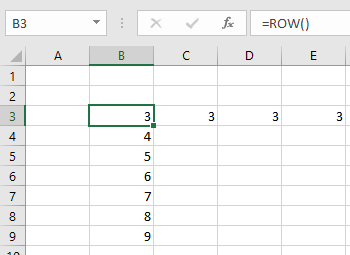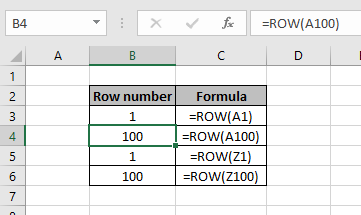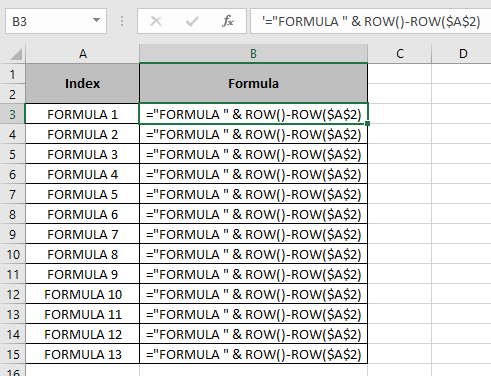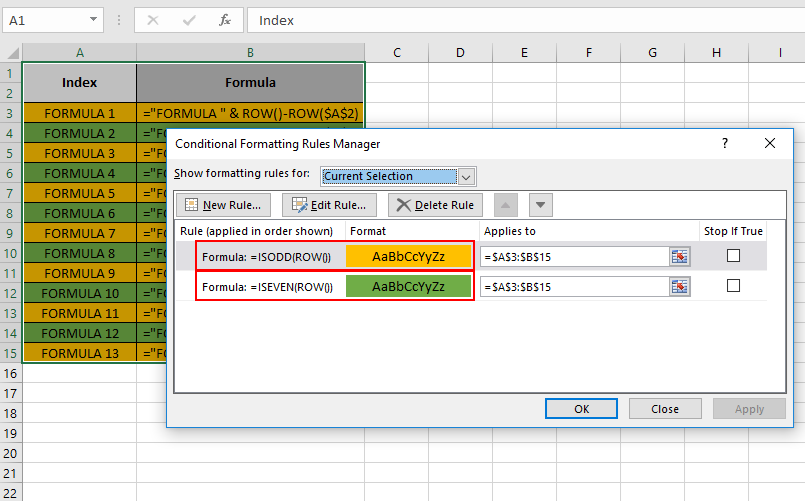# How to use the ROW function in Microsoft Excel

The ROW function returns the row number of the cell or the cell reference. It returns N if the cell or cell reference is cN. c can be any column index.
Syntax:

= ROW ([cell_reference])

Let’s run some tests on using the ROW function Excel

1. If no argument is passed in the function, then the function returns the row number of the cell.1. If any cell is refereed in the argument, then the function returns the row number of the refereed cell.1. It is used to label the Row index in the data.In the above snapshot, the same formula is applied in each cell.

1. It is most widely used in Conditional formatting to highlight the rows in the data.As you can see from the above examples that how to use ROW function in Excel.

Hope you understood how to use the ROW function in Excel. Explore more articles on Excel cell refrence function here. Please state your queries in the comment box below.

Related Articles:

Excel COLUMN Function in Excel

How to use the ROWS function in Excel

How To Switch Rows and Columns in Excel

Popular Articles:

50 Excel Shortcuts to Increase Your Productivity

How to use the VLOOKUP Function in Excel

How to use the COUNTIF function in Excel 2016

1.